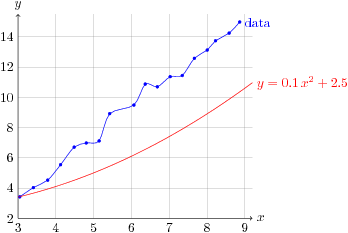October 19, 2021

# Creating plots in LaTeX with Gnuplot and Tikz

Plotting data in LaTeX is a useful thing to do. Xavier Delaunay shows how to do it on his homepage:

http://d.xav.free.fr/tikz/index.html

The plot looks like this:The advantage of using Tikz to draw plots is, that things like fonts and arrow-heads will be the same in the document and in the figures.

Below I've replicated Xavier Delaunays LaTeX code, in the unlikely case that his site goes offline (Xavier, hope you don't mind).

\documentclass{article} \usepackage{tikz}   \begin{document} \pagestyle{empty}   \begin{tikzpicture}[x=1cm,y=0.4cm]   \def\xmin{3} \def\xmax{9.2} \def\ymin{2} \def\ymax{15.5}   % grid \draw[style=help lines, ystep=2, xstep=1] (\xmin,\ymin) grid (\xmax,\ymax);   % axes \draw[->] (\xmin,\ymin) -- (\xmax,\ymin) node[right] {$x$}; \draw[->] (\xmin,\ymin) -- (\xmin,\ymax) node[above] {$y$};   % xticks and yticks \foreach \x in {3,4,...,9} \node at (\x, \ymin) [below] {\x}; \foreach \y in {2,4,...,14} \node at (\xmin,\y) [left] {\y};   % plot the data from the file data.dat % smooth the curve and mark the data point with a dot \draw[color=blue] plot[smooth,mark=*,mark size=1pt] file {data.dat} node [right] {data};   % generate and plot another a curve y = 0.1 x^2 + 2.5 % this generates the files figure.parabola.gnuplot and figure.parabola.table \draw[color=red, domain=\xmin:\xmax] plot[id=parabola] function{0.1*x**2 + 2.5} node [right] {$y=0.1\,x^2 + 2.5$};   \end{tikzpicture}   \end{document}

The data.dat file is simply a tab-separated file with$(x,y)$ coordinates#### kostas

View all posts by kostas →

This site uses Akismet to reduce spam. Learn how your comment data is processed.